Courses

# Polarization NAT

## 10 Questions MCQ Test Topic wise Tests for IIT JAM Physics | Polarization NAT

Description
This mock test of Polarization NAT for Physics helps you for every Physics entrance exam. This contains 10 Multiple Choice Questions for Physics Polarization NAT (mcq) to study with solutions a complete question bank. The solved questions answers in this Polarization NAT quiz give you a good mix of easy questions and tough questions. Physics students definitely take this Polarization NAT exercise for a better result in the exam. You can find other Polarization NAT extra questions, long questions & short questions for Physics on EduRev as well by searching above.
*Answer can only contain numeric values
QUESTION: 1

### Unpolarized light of intensity. I0,  is incident on a series of 3 polarizing filters, the axis of the second filter is oriented at 45° to that of the first filter, while the axis of the third filter is oriented at 90° to that of the first filter. What fraction of the incident light that is transmitted through the third filter?

Solution:

Light through the first filter is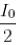I0= Incident intensity.
Through the second filter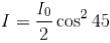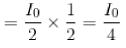Through the third filter, intensity is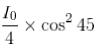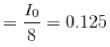*Answer can only contain numeric values
QUESTION: 2

### The critical angle of light in a certain substance is 45°. What is the polarising angle?

Solution:

Solution :- If  'n' is refractive index and ic is critical velocity,

n = 1/Sin ic = 1 / Sin 45 = 1.414

Now, According to Brewster's law , if  ' ip ' is polarising angle  then

n = tan ip

So , ​tan ip  = 1.414

i.e. ​ ip =  54.70

*Answer can only contain numeric values
QUESTION: 3

### Unpolarized light is incident on two ideal polarizers in series, the polarizers are oriented so that no light emerges through the second polarizer. A third polarizer is now inserted between the first two and its orientation direction is continuously rotated through 180°. What is the maximum fraction of the incident power transmitted through all three polarizers?

Solution:

Let incident intensity be I
Intensity after first polarizer =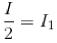Intensity after the second polarizer = I1 cosØ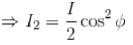where Ø s the angle between the polarizer pass axis of the second polarizer and first polarizer
Intensity of light through the 3rd polarizer is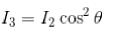Intensity of light through the 3rd polarizer is I= I2 cos2 θ
where θ is the angle between pass axis polarizer 2 and 3. For I3 to be maximum.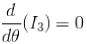Put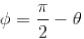because
the first and third polarizers are orthogonal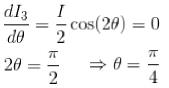Put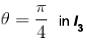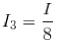Fraction is 0.125

*Answer can only contain numeric values
QUESTION: 4

The critical angle of a medium is 45° Find the polarizing angle.

Solution: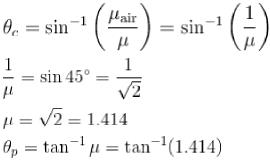= 54.75°

*Answer can only contain numeric values
QUESTION: 5

A beam of light polarized in the x direction is travelling in the z direction. It passess through a polarizer, travels some distance, and reaches an analyser you can orient the pass axis of the analyzer only in the x or the y direction. You find that the intensity is three times smaller when the pass axis of the analyser is in the y direction than it was when the pass axis was in the x direction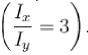Find the angle between the pass axis of the polarizer and the x-axis (in degrees).

Solution:

If θ is the angle of polarizer’s pass axis with the x-axis and / is the intensity of the light beam between the polarizer and the analyzer.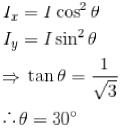*Answer can only contain numeric values
QUESTION: 6

Unpolarized light is incident on a pair of ideal linear polarizers whose transmission axis make an angle of 45° with each other, the transmitted light intensity through both polarizers in what percentage of the incident intensity?

Solution:

Light incident = l0
Intensity of light through first polarizer =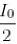Intensity of light through second polarizer =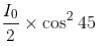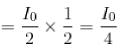Percentage =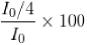= 25%

*Answer can only contain numeric values
QUESTION: 7

A beam of light polarized in the x direction is travelling in the z direction. It passess through a polarizer, travels some distance, and reaches an analyser you can orient the pass axis of the analyzer only in the x or the y direction. You find that the intensity is three times smaller when the pass axis of the analyser is in the y direction than it was when the pass axis was in the x direction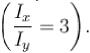Suppose that the original light beam were polarized in the / direction, everything else remaining the same. What value of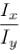would you observe?

Solution:

Using the polarized light would change the beam intensity / between the polarizer and analyser but it would not change the ratio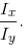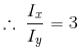*Answer can only contain numeric values
QUESTION: 8

The refractive indices of glass and water are 1.54 and 1.33. Which will be greater: the polarising angle for a beam incident from water to glass or that for a beam incident from glass to water?

Solution:

By Brewster’s law, the polarising angle p is given by
tan p = μ,
Where μ  is the refractive index of the second medium with respect to the first.
For water to glass,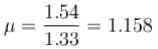p = tan-1 (1.158) = 49.2°
For glass to water,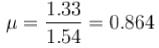p = tan-1 (0.864) = 40.8°
Thus, p is larger from water to glass.

*Answer can only contain numeric values
QUESTION: 9

If the polarizing angle of a medium is 60°. Then find the angle of refraction, (in degrees)

Solution:

Polarizing angle is the angle of incidence for which the refracted and reflected rays are orthogonal.
θi = 60°
⇒ θreflected = 60°
⇒ θreflected = 90°- 60° = 30°